• 做工程的免不了要做资料算量，Excel是工作中最常用的工具之一。只要搞清楚它的一些使用小技巧，工作效率那是嗖嗖的往上蹭啊。下面这些，你就绝对不能错过！一、数字处理1、取绝对值=ABS(数字)2、取整=INT(数字)3、四...


做工程的免不了要做资料算量，Excel是工作中最常用的工具之一。只要搞清楚它的一些使用小技巧，工作效率那是嗖嗖的往上蹭啊。下面这些，你就绝对不能错过！一、数字处理1、取绝对值=ABS(数字)2、取整=INT(数字)3、四舍五入=ROUND(数字,小数位数)二、判断公式1、把公式产生的错误值显示为空公式：C2=IFERROR(A2/B2,"")说明：如果是错误值则显示为空，否则正常显示。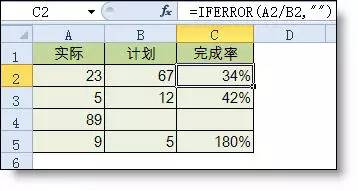2、IF多条件判断返回值公式：C2=IF(AND(A2<500,B2="未到期"),"补款","")说明：两个条件同时成立用AND,任一个成立用OR函数。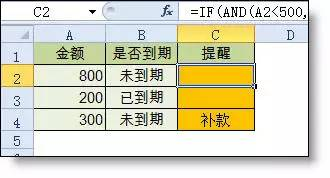三、统计公式1、统计两个表格重复的内容公式:B2=COUNTIF(Sheet15!A:A,A2)说明：如果返回值大于0说明在另一个表中存在，0则不存在。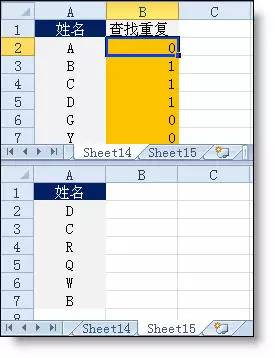2、统计不重复的总人数公式：C2=SUMPRODUCT(1/COUNTIF(A2:A8,A2:A8))说明:用COUNTIF统计出每人的出现次数，用1除的方式把出现次数变成分母，然后相加。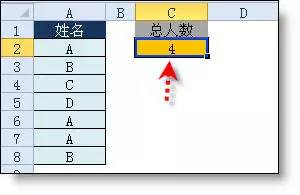四、求和公式1、隔列求和公式：H3=SUMIF($A$2:$G$2,H$2,A3:G3)或=SUMPRODUCT((MOD(COLUMN(B3:G3),2)=0)*B3:G3)说明：如果标题行没有规则用第2个公式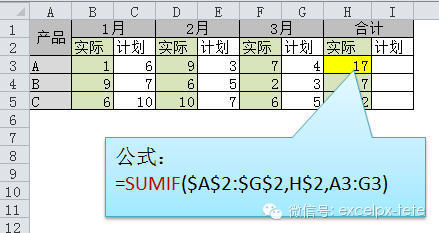2、单条件求和公式：F2=SUMIF(A:A,E2,C:C)说明：SUMIF函数的基本用法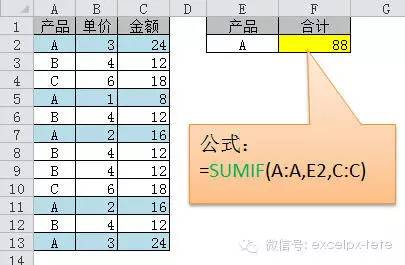3、单条件模糊求和公式：详见下图说明：如果需要进行模糊求和，就需要掌握通配符的使用，其中星号是表示任意多个字符，如"*A*"就表示a前和后有任意多个字符，即包含A。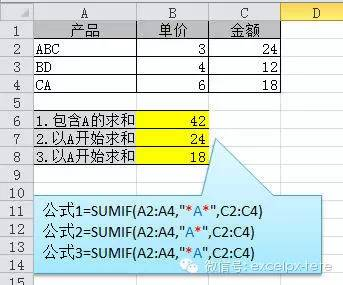4、多条件模糊求和公式：C11=SUMIFS(C2:C7,A2:A7,A11&"*",B2:B7,B11)说明：在sumifs中可以使用通配符*5、多表相同位置求和公式：b2=SUM(Sheet1:Sheet19!B2)说明：在表中间删除或添加表后，公式结果会自动更新。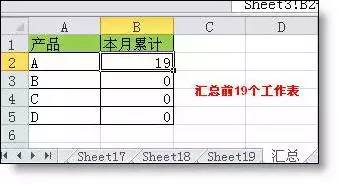6、按日期和产品求和公式：F2=SUMPRODUCT((MONTH($A$2:$A$25)=F$1)*($B$2:$B$25=$E2)*$C$2:$C$25)说明：SUMPRODUCT可以完成多条件求和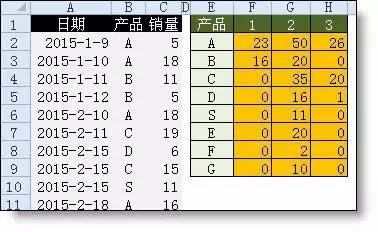五、查找与引用公式1、单条件查找公式公式1：C11=VLOOKUP(B11,B3:F7,4,FALSE)说明：查找是VLOOKUP最擅长的，基本用法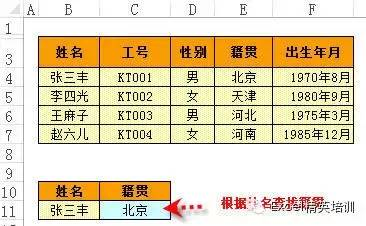2、双向查找公式公式：=INDEX(C3:H7,MATCH(B10,B3:B7,0),MATCH(C10,C2:H2,0))说明：利用MATCH函数查找位置，用INDEX函数取值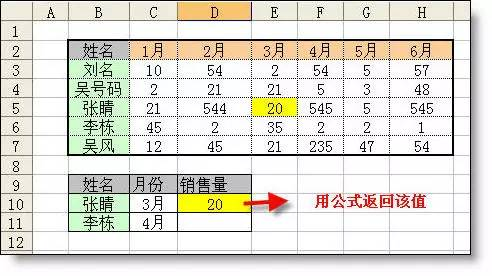3、查找最后一条符合条件的记录。公式：详见下图说明：0/(条件)可以把不符合条件的变成错误值，而lookup可以忽略错误值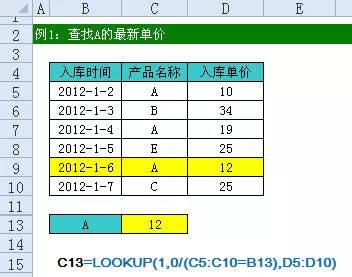4、多条件查找公式:详见下图说明:公式原理同上一个公式5、指定区域最后一个非空值查找公式;详见下图说明：略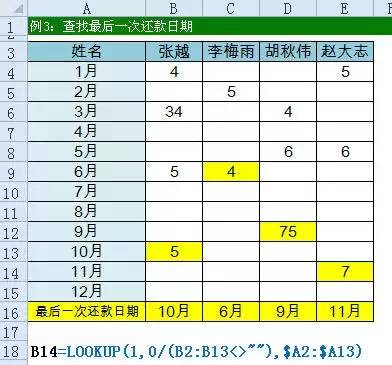6、按数字区域间取对应的值公式：详见下图公式说明：VLOOKUP和LOOKUP函数都可以按区间取值，一定要注意，销售量列的数字一定要升序排列。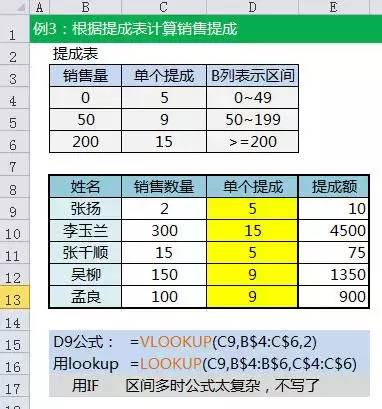六、字符串处理公式1、多单元格字符串合并公式：c2=PHONETIC(A2:A7)说明：Phonetic函数只能对字符型内容合并，数字不可以。2、截取除后3位之外的部分公式：=LEFT(D1,LEN(D1)-3)说明：LEN计算出总长度,LEFT从左边截总长度-3个3、截取-前的部分公式:B2=Left(A1,FIND("-",A1)-1)说明：用FIND函数查找位置，用LEFT截取。4、截取字符串中任一段的公式公式:B1=TRIM(MID(SUBSTITUTE($A1," ",REPT(" ",20)),20,20))说明:公式是利用强插N个空字符的方式进行截取5、字符串查找公式：B2=IF(COUNT(FIND("河南",A2))=0,"否","是")说明: FIND查找成功，返回字符的位置，否则返回错误值，而COUNT可以统计出数字的个数，这里可以用来判断查找是否成功。6、字符串查找一对多公式：B2=IF(COUNT(FIND({"辽宁","黑龙江","吉林"},A2))=0,"其他","东北")说明：设置FIND第一个参数为常量数组，用COUNT函数统计FIND查找结果七、日期计算公式1、两日期相隔的年、月、天数计算A1是开始日期(2011-12-1)，B1是结束日期(2013-6-10)。计算：相隔多少天？=datedif(A1,B1,"d") 结果：557相隔多少月? =datedif(A1,B1,"m") 结果：18相隔多少年? =datedif(A1,B1,"Y") 结果：1不考虑年相隔多少月？=datedif(A1,B1,"Ym") 结果：6不考虑年相隔多少天？=datedif(A1,B1,"YD")  结果：192不考虑年月相隔多少天？=datedif(A1,B1,"MD") 结果：9datedif函数第3个参数说明："Y" 时间段中的整年数。"M" 时间段中的整月数。"D" 时间段中的天数。"MD" 天数的差。忽略日期中的月和年。"YM" 月数的差。忽略日期中的日和年。"YD" 天数的差。忽略日期中的年。2、扣除周末天数的工作日天数公式：C2=NETWORKDAYS.INTL(IF(B2说明：返回两个日期之间的所有工作日数，使用参数指示哪些天是周末，以及有多少天是周末。周末和任何指定为假期的日期不被视为工作日。(来源：网络，版权归原作者)猜您喜欢◆  超有用的15个EXCEL公式◆  10个让你“事半功倍”的Excel函数◆  Excel 用得“巧”，工作起来没烦恼！分享 · 共赢电气圈，一个有态度的圈子展开全文• 点击上方△蓝字可关注我们会计人2019职场充电站坚持阅读... Excel常用快捷键 8大类常用公式 一.数字处理1取绝对值=ABS(数字)2数字取整=INT(数字)3数字四舍五入=ROUND(数字,小数位数)二.判断公式4把公式返回的错误...


点击上方△蓝字可关注我们会计人2019职场充电站坚持阅读，必遇见更优秀的自己只会Ctrl+C和Ctrl+V，怎么面对职场挑战？练就Excel无影手，关键就是TA！戳图学习，新技能get√！    Excel最常用快捷键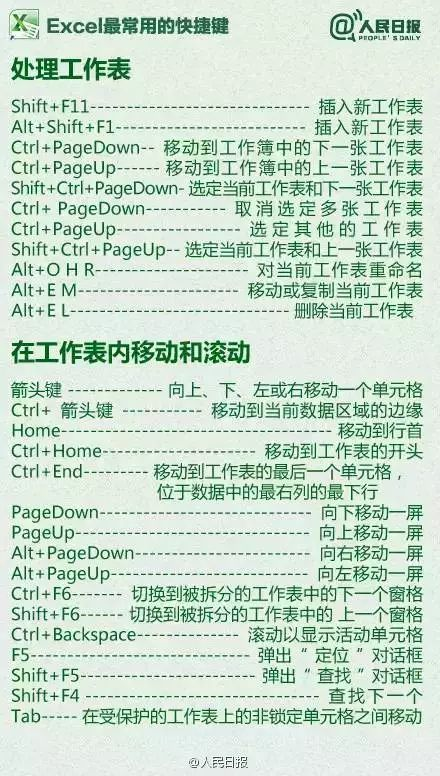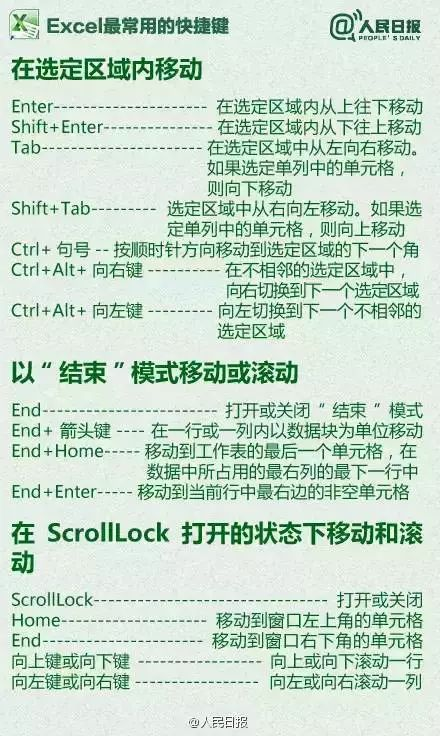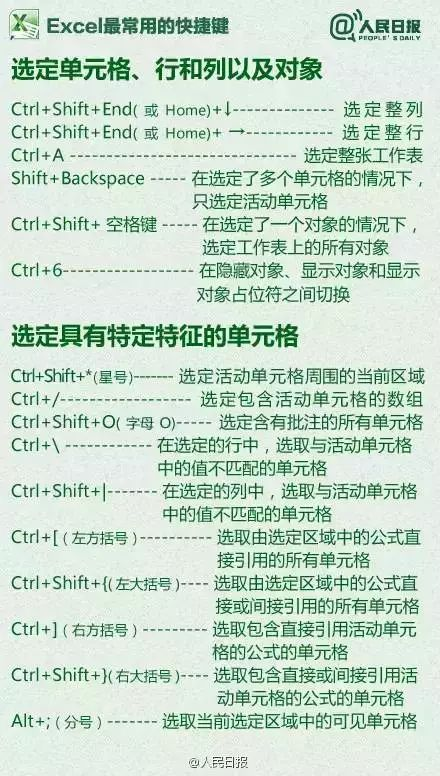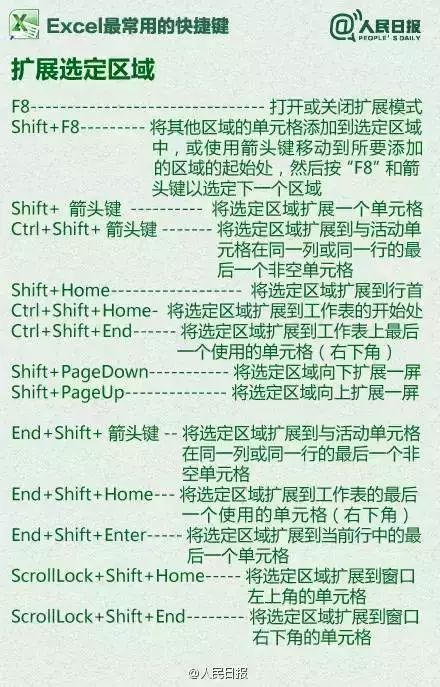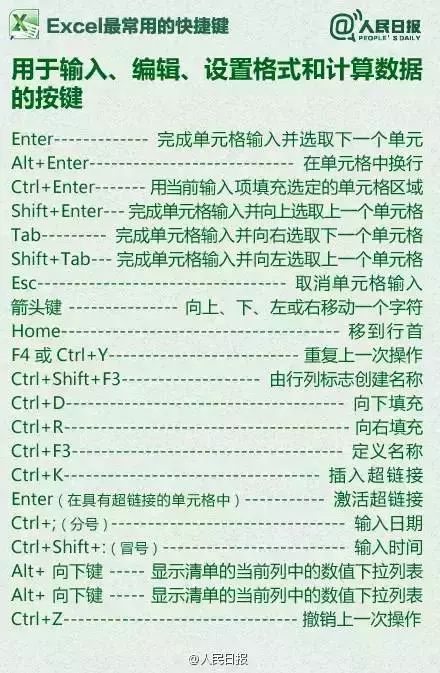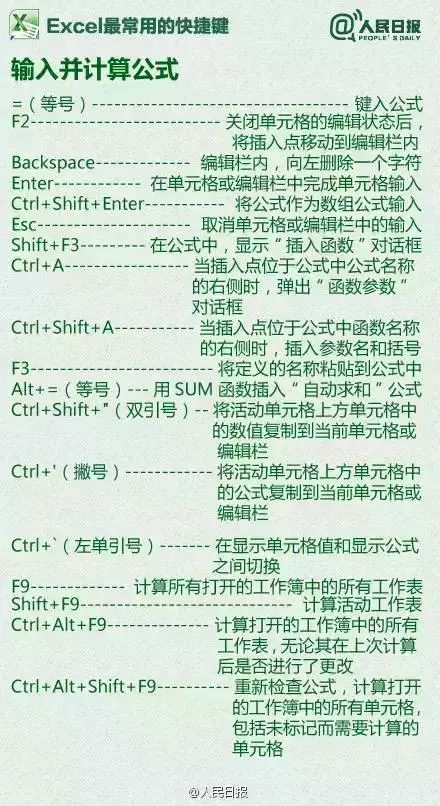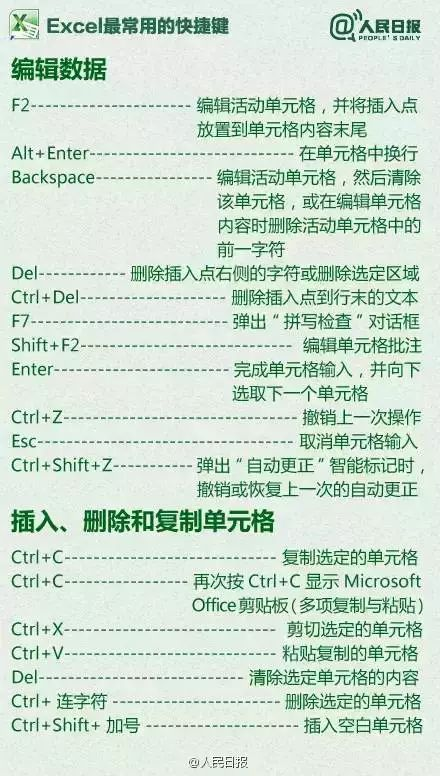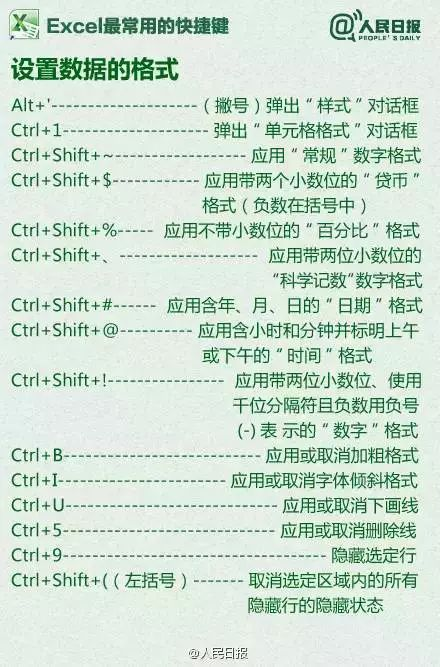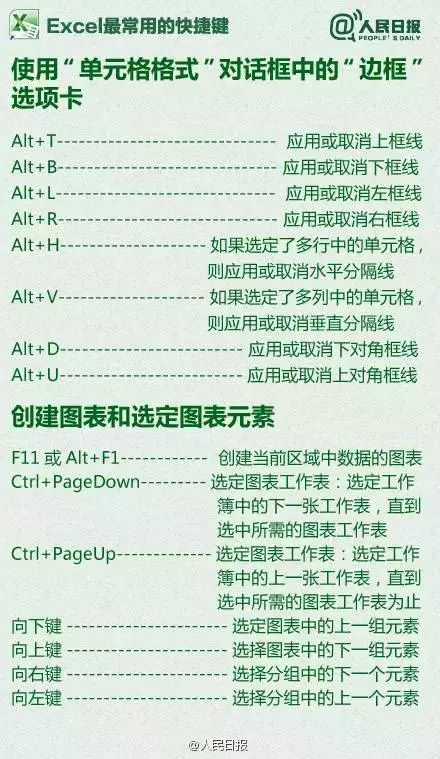8大类常用公式   一.数字处理1取绝对值=ABS(数字)2数字取整=INT(数字)3数字四舍五入=ROUND(数字,小数位数)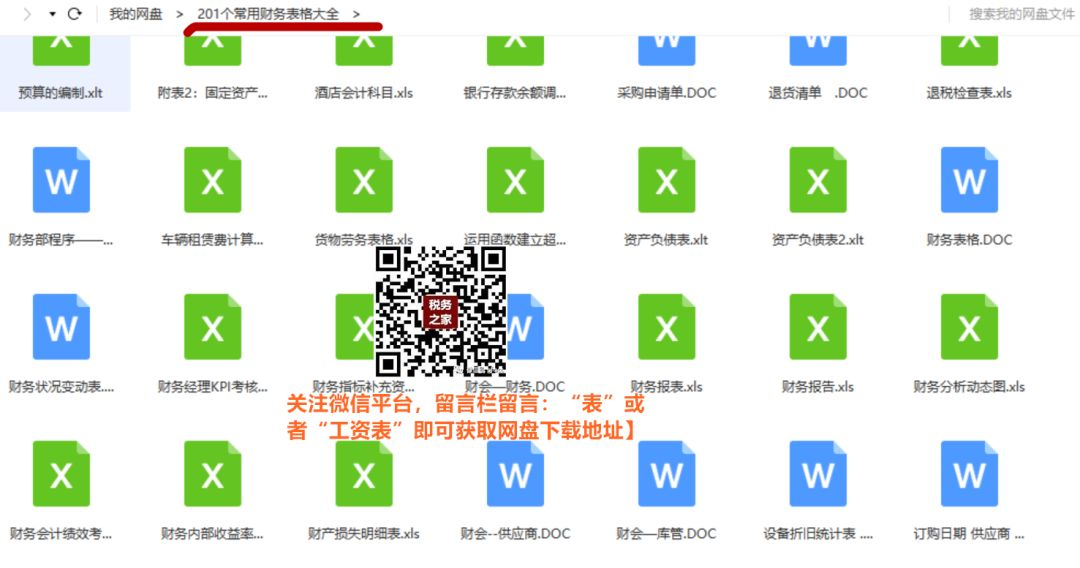二.判断公式4把公式返回的错误值显示为空公式：C2=IFERROR(A2/B2,"")说明：如果是错误值则显示为空，否则正常显示。5IF的多条件判断公式：C2=IF(AND(A2<500,B2="未到期"),"补款","")说明：两个条件同时成立用AND,任一个成立用OR函数。三.统计公式6统计两表重复公式:B2=COUNTIF(Sheet15!A:A,A2)说明：如果返回值大于0说明在另一个表中存在，0则不存在。7统计年龄在30~40之间的员工个数=FREQUENCY(D2:D8,{40,29})8统计不重复的总人数公式：C2=SUMPRODUCT(1/COUNTIF(A2:A8,A2:A8))说明:用COUNTIF统计出每人的出现次数，用1除的方式把出现次数变成分母，然后相加。9按多条件统计平均值F2公式=AVERAGEIFS(D:D,B:B,"财务",C:C,"大专")10中国式排名公式=SUMPRODUCT(($D$4:$D$9>=D4)*(1/COUNTIF(D$4:D$9,D$4:D$9)))四.求和公式11隔列求和公式：H3=SUMIF($A$2:$G$2,H$2,A3:G3)或=SUMPRODUCT((MOD(COLUMN(B3:G3),2)=0)*B3:G3)说明：如果标题行没有规则用第2个公式12单条件求和公式：F2=SUMIF(A:A,E2,C:C)说明：SUMIF函数的基本用法13单条件模糊求和公式：详见下图说明：如果需要进行模糊求和，就需要掌握通配符的使用，其中星号是表示任意多个字符，如"*A*"就表示a前和后有任意多个字符，即包含A。14多条求模糊求和公式：C11=SUMIFS(C2:C7,A2:A7,A11&"*",B2:B7,B11)说明：在sumifs中可以使用通配符*15多表相同位置求和公式：b2=SUM(Sheet1:Sheet19!B2)说明：在表中间删除或添加表后，公式结果会自动更新。16按日期和产品求和公式：F2=SUMPRODUCT((MONTH($A$2:$A$25)=F$1)*($B$2:$B$25=$E2)*$C$2:$C$25)说明：SUMPRODUCT可以完成多条件求和五.查找与引用公式17单条件查找公式1：C11=VLOOKUP(B11,B3:F7,4,FALSE)说明：查找是VLOOKUP最擅长的，基本用法18双向查找公式：=INDEX(C3:H7,MATCH(B10,B3:B7,0),MATCH(C10,C2:H2,0))说明：利用MATCH函数查找位置，用INDEX函数取值19查找最后一个符合条件记录公式：详见下图说明：0/(条件)可以把不符合条件的变成错误值，而lookup可以忽略错误值20多条件查找公式:详见下图说明:公式原理同上一个公式21指定非空区域最后一个值查找公式;详见下图说明：略22区间取值公式：详见下图公式说明：VLOOKUP和LOOKUP函数都可以按区间取值，一定要注意，销售量列的数字一定要升序排列。六.字符串处理公式23多单元格字符合并公式：c2=PHONETIC(A2:A7)说明：Phonetic函数只能对字符型内容合并，数字不可以。24截取除后3位之外的部分公式：=LEFT(D1,LEN(D1)-3)说明：LEN计算出总长度,LEFT从左边截总长度-3个25截取 - 之前的部分公式:B2=Left(A1,FIND("-",A1)-1)说明：用FIND函数查找位置，用LEFT截取。26截取字符串中任一段公式:B1=TRIM(MID(SUBSTITUTE($A1," ",REPT(" ",20)),20,20))说明:公式是利用强插N个空字符的方式进行截取27字符串查找公式：B2=IF(COUNT(FIND("河南",A2))=0,"否","是")说明: FIND查找成功，返回字符的位置，否则返回错误值，而COUNT可以统计出数字的个数，这里可以用来判断查找是否成功。28字符串查找一对多公式：B2=IF(COUNT(FIND({"辽宁","黑龙江","吉林"},A2))=0,"其他","东北")说明：设置FIND第一个参数为常量数组，用COUNT函数统计FIND查找结果七.日期计算公式29两日期间隔的年、月、日计算A1是开始日期(2011-12-1)，B1是结束日期(2013-6-10)。计算：相隔多少天？=datedif(A1,B1,"d") 结果：557相隔多少月? =datedif(A1,B1,"m") 结果：18相隔多少年? =datedif(A1,B1,"Y") 结果：1不考虑年相隔多少月？=datedif(A1,B1,"Ym") 结果：6不考虑年相隔多少天？=datedif(A1,B1,"YD") 结果：192不考虑年月相隔多少天？=datedif(A1,B1,"MD") 结果：9datedif函数第3个参数说明："Y" 时间段中的整年数。"M" 时间段中的整月数。"D" 时间段中的天数。"MD" 天数的差。忽略日期中的月和年。"YM" 月数的差。忽略日期中的日和年。"YD" 天数的差。忽略日期中的年。30扣除周末的工作日天数公式：C2=NETWORKDAYS.INTL(IF(B2说明：返回两个日期之间的所有工作日数，使用参数指示哪些天是周末，以及有多少天是周末。周末和任何指定为假期的日期不被视为工作日八.其他常用公式31创建工作表目录的公式把所有的工作表名称列出来，然后自动添加超链接，管理工作表就非常方便了。使用方法：第1步：在定义名称中输入公式：=MID(GET.WORKBOOK(1),FIND("]",GET.WORKBOOK(1))+1,99)&T(NOW())第2步、在工作表中输入公式并拖动，工作表列表和超链接已自动添加=IFERROR(HYPERLINK("#'"&INDEX(Shname,ROW(A1))&"'!A1",INDEX(Shname,ROW(A1))),"")32中英文互译公式=FILTERXML(WEBSERVICE("http://fanyi.youdao.com/translate?&i="&A2&"&doctype=xml&version"),"//translation") excel中的函数公式千变万化，今天就整理这么多了。如果你能掌握一半，在工作中也基本上遇到不难题了。来源|人民日报/excel精英培训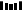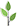关注我们您得到的不仅是财务专业知识还有优质财务圈人脉以及职业发展的契机


展开全文• 今天和大家分享一组常用函数公式的使用方法，用心掌握这些函数，工作效率会大大提升。欢迎转发给更多有需要的人。一、相关概念(一)函数语法由函数名+括号+参数组成例： 求和函数：SUM(A1,B2,…) 。参数与参数之 间用...
今天和大家分享一组常用函数公式的使用方法，用心掌握这些函数，工作效率会大大提升。欢迎转发给更多有需要的人。一、相关概念(一)函数语法由函数名+括号+参数组成例： 求和函数：SUM(A1,B2,…) 。参数与参数之 间用逗号“,”隔开(二)运算符1. 公式运算符：加(+)、减(-)、乘(*)、除(/)、百分号(%)、乘幂(＾) 2. 比较运算符：大与(>)、小于(=) 、不等于(<>)3. 引用运算符：区域运算符(：)、联合运算符(,)(三)单元格的相对引用与绝对引用例： A1 $A1 锁定第A列 A$1 锁定第1行 $A$1 锁定第A列与第1行二、常用函数(一)数学函数1. 求和 =SUM(数值1,数值2,……)2. 条件求和 =SUMIF(查找的范围,条件(即对象),要求和的范围)例：(1)=SUMIF(A1:A4,”>=200”,B1:B4) 函数意思：对第A1栏至A4栏中，大于等于200的数值对应的第B1列至B4列中数值求和(2)=SUMIF(A1:A4,”<300”,C1:C4)函数意思：对第A1栏至A4栏中，小于300的数值对应的第C1栏至C4栏中数值求和3. 求个数 =COUNT(数值1,数值2,……)例：(1) =COUNT(A1:A4) 函数意思：第A1栏至A4栏求个数 (2) =COUNT(A1:C4) 函数意思：第A1栏至C4栏求个数4. 条件求个数 =COUNTIF(范围,条件)例：(1) =COUNTIF(A1:A4，”<>200”) 函数意思：第A1栏至A4栏中不等于200的栏求个数 (2) =COUNTIF(A1:C4,”>=1000”) 函数意思：第A1栏至C4栏中大于等1000的栏求个数5. 求算术平均数 =AVERAGE(数值1,数值2,……)例：(1) =AVERAGE(A1,B2)(2) =AVERAGE(A1:A4)6. 四舍五入函数 =ROUND(数值,保留的小数位数)7. 排位函数 =RANK(数值,范围,序别) 1-升序 0-降序例：(1) =RANK(A1,A1:A4,1) 函数意思：第A1栏在A1栏至A4栏中按升序排序，返回排名值。 (2) =RANK(A1,A1:A4,0) 函数意思：第A1栏在A1栏至A4栏中按降序排序，返回排名值。8. 乘积函数 =PRODUCT(数值1,数值2,……)9. 取绝对值 =ABS(数字)10. 取整 =INT(数字)(二)逻辑函数条件函数：=IF(条件,执行条件,执行条件假)—可执行七层嵌套例： A1=5 A2=3 A3=6 A4=7=IF(A1>A2,1,0)=1(真)=IF(A1=IF(A1>A2,IF(A3>A4,8,9),1)=9(三)文本函数1. 截取函数： =LEFT(文本,截取长度)—从左边截取=RIGHT(文本,截取长度)—从右边截取=MID(文本,开始位,截取长度)—从中间截取2. 计算字符长度：LEN(文本)—文本的长度3. 合并字符函数：CONCATENATE(文本1,文本2,…)4. 在字符串中查找特定字符：FIND(文本,范围,数值)—数值表示查找第几个=FIND(“a”,“abcadeafga”,2)=4=FIND(“a”,“abcadeafga”,3)=7=FIND(“a”,“abcadeafga”,4)=105. 比较两个字符是否完全相符：EXACT(文本1,文本2)=EXACT(“a”,“a”)=TRUE=EXACT(“a”,“ab”)=FALSE=EXACT(“a”,“A”)=FALSE6. 将数值转化为文本：TEXT(数值,参数) )—参数一般为07. 将数值型字符转换为数值：VALUE(数值文本)(四)判断公式1. 把公式产生的错误显示为空公式：C2=IFERROR(A2/B2,””)说明：如果是错误值则显示为空，否则正常显示。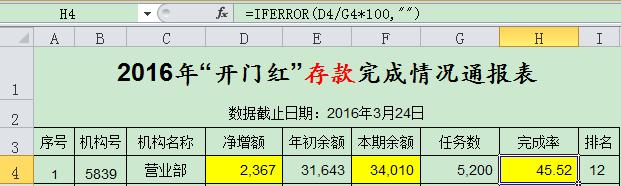2. IF多条件判断返回值公式：C2=IF(AND(A2<500,B2=”未到期”),”补款”,””)说明：两个条件同时成立用AND，任一个成立用OR函数。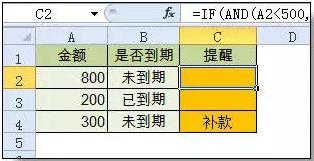(五)统计公式1. 统计两个表格重复的内容公式：B2=COUNTIF(Sheet15!A:A,A2)说明：如果返回值大于0说明在另一个表中存在，0则不存在。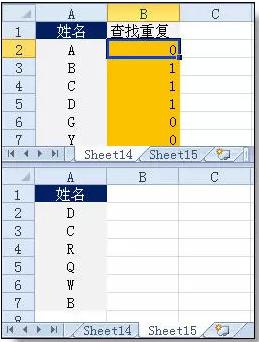2. 统计不重复的总人数公式：C2=SUMPRODUCT(1/COUNTIF(A2:A8,A2:A8))说明：用COUNTIF统计出每人的出现次数，用1除的方式把出现次数变成分母，然后相加。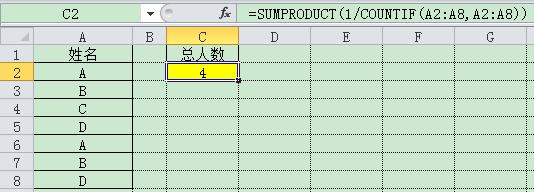(六)求和公式1. 隔列求和公式：H3=SUMIF($A$2: $G$2,H$2,A3:G3)或者： =SUMPRODUCT((MOD(COLUMN(B3:G3),2)=0)*B3:G3)说明：如果标题行没有规则用第2个公式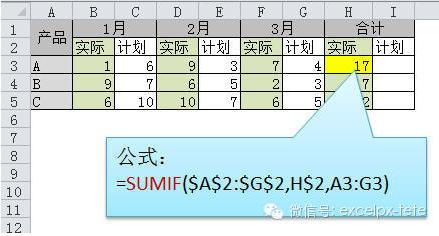2. 单条件求和3. 单条件模糊求和4. 多条件模糊求和5. 多表相同位置求和公式：B2=SUM(Sheet1:Sheet19!B2)说明：在表中间删除或添加表后，公式结果会自动更新。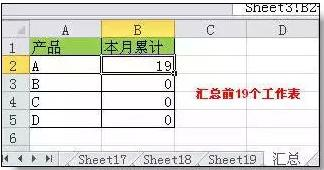6. 按日期和产品求和(七)查找与引用公式1. 单条件查找公式公式1： C11=VLOOKUP(B11,B3:F7,4,FALSE)说明：查找是VLOOKUP最擅长的，基本用法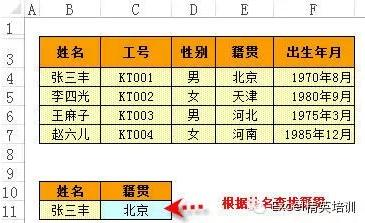2. 双向查找公式公式=INDEX(C3:H7,MATCH(B10,B3:B7,0),MATCH(C10,C2:H2,0))说明：利用MATCH函数查找位置，用INDEX函数取值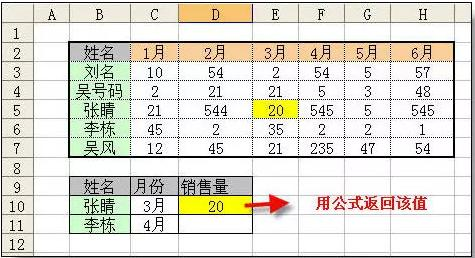3. 查找最后一条符合条件的记录。公式：详见下图说明：0/(条件)可以把不符合条件的变成错误值，而lookup可以忽略错误值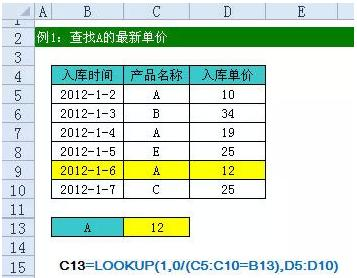4. 多条件查找公式：详见下图说明：公式原理同上一个公式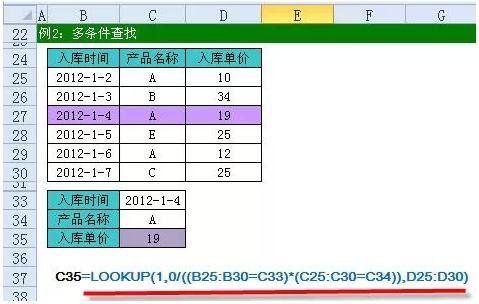5. 指定区域最后一个非空值查找6. 按数字区域间取对应的值 展开全文• excel函数公式大全及使用方法请教EXCEL函数的应用, 麻烦大家告诉仔细点,.15个常用的Excel函数公式，拿来即用查找重复内容｜=IF(COUNTIF(A:A,A2)>1,"重复","")｜ ｜重复内容首次出现时不提示｜=IF(COUNTIF(A$2:A2,...
Excel里的普通常用函数公式有哪些？excel函数公式大全及使用方法请教EXCEL函数的应用, 麻烦大家告诉仔细点,.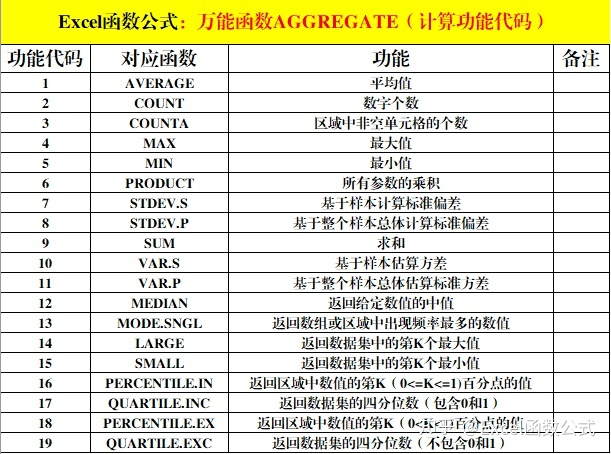15个常用的Excel函数公式，拿来即用查找重复内容｜=IF(COUNTIF(A:A,A2)>1,"重复","")｜ ｜重复内容首次出现时不提示｜=IF(COUNTIF(A$2:A2,A2)>1,"重复","")｜ ｜重复内容首次出现时提示重复｜=IF(COUNTIF(A2:A99,A2)>1,"重复","")｜excel中常用函数有哪些excel最常用的八个函数分别是哪些？SUM函数 SUM函数的作用是CSS布局HTML小编今天和大家分享和。 统计一个单元格区域： =sum(A1:A10) 统计多个单元格区域： =sum(A1:A10,C1:C10)AVERAGE函数 Average 的作用是计算平均数。COUNT函数 COUNT函数计算含有数字的单元格的个数。EXCEL最常用的函数有哪些？Excel常用的函数计算公式大全(一看就会)计算机等级考试=公式名称(参数1，参数2，。。。。。)=sum(计算范围)=average(计算范围)=sumifs(CSS布局HTML小编今天和大家分享和范围，条件范围1，符合条件1，条件范围2，符合条件2，。。。。。。)=vlookup(翻译对象，到哪里翻译，显示哪一种，精确匹配)=rank(对谁排名。Excel 函数的加减乘除公式各是什么？还有，除了加减乘除公式外，excel里还有什么公式需要记住？还有，excelexcel中公式的运用，常见的加减乘除公式如下：加法公式：“=SUM(第一个加数:最后一个加数)”；减法公式：“=被减数-减数”；除法公式：“=被除数/除数”。 具体应用如下：excel函数公式中，如何计算“个数”？重复内容首次出现时不提示 =IF(COUNTIF(A$2:A2,A2)>1,"重复","")重复内容首次出现时提示重复 =IF(COUNTIF(A2:A99,A2)>1,"重复","")根据出生年月计算年龄 =DATEDIF(A2,TODAY(),"y")
展开全文• 下面是学习啦小编为您精心整理的最常用Excel函数公式大全。篇一零、随机数1、随机数函数：=RAND()首先介绍一下如何用RAND()函数来生成随机数(同时返回多个值时是不重复的)。RAND()函数返回的随机数字的范围是大于0...
• 最近后台的留言实在是太多了，由于时间关系，没办法一一给小伙伴们解答，所以这里我们总结了小伙伴们问的最多的问题，整理出了10个职场人士最常用excel公式，希望能帮大家排忧解难，赶紧来看看吧!​＊＊＊＊＊＊＊...
• Excel中的数据统计分析，离不开函数公式，相对于一些“高大上”的应用技巧，函数，公式是基础，也是灵魂。一、求和。(一)单条件求和。函数：Sumif。功能：对满足条件的单元格求和。语法结构：=Sumif(条件范围,条件,...
• 助手反馈每天都会接到关于各种多条件查询的问题，为了帮大家彻底解决这类难题，干脆整理出一篇Excel办公常用多条件查询函数公式大全，方便大家自学备查！下面是问题场景和效果演示，动图如下所示。下文会给出多种...es 多条件查询 es多条件查询
• 下面是21个常用Excel函数公式的用法，学会这些套路，让工作效率再提高一些。建议收藏！1、IF函数条件判断IF函数是最常用的判断类函数之一，能完成非此即彼的判断。如下图，考核得分的标准为9分，要判断B列的考核...excel减法函数
• 作者：祝洪忠 转自：Excel之家ExcelHome小伙伴们好啊，今天老祝和大家一起学习一个Excel函数公式中的常用技巧——锁头不锁尾。先来看下面这个表格，要计算从一月份开始，到当前月份的累计销量：C2单元格可以输入以下...
• 目录一、数字处理1、取绝对值2、取整3、四舍五入二、判断公式1、把公式产生的错误值显示为空2、IF多条件判断返回值三、统计公式1、统计两个表格重复的内容2、统计不重复的总人数四、求和公式1、隔列求和2、单条件...
• excel表格时间和日期应用 – Excel常用函数公式及技巧自动显示当前日期公式=YEAR(NOW()) 当前年=MONTH(NOW()) 当前月=DAY((NOW())) 当前日如何在单元格中自动填入当前日期Ctrl+;如何判断某日是否星期天=WEEKDAY(A2,2...
• 有网友问到excel中能不能用函数求和，excel函数是很强大的功能，求和就不在话下了，在常用的Excel操作中，SUM求和是使用频率最高的一个函数。那Excel求和公式怎么用?今天，小编就和大家说一下Excel求和公式的使用...excel一列求和 excel保存快捷键
• 单元格地址引用1、相对引用、绝对引用、混合引用，快捷键F4；例如多列变一列、一列变多列的相对引用，增长率的相对引用，本利和的混合...5、F9功能键逐步解析函数：选中需要解释的部分-F9,会出来这个函数的运行结...
• 每天一篇原创Excel图文微信公众号：AhaExcelNO.68-什么是函数公式作者：看见星光微博：EXCELers / 知识星球：ExcelHI，我是星光，咱们今天来聊点Excel的基础知识——什么是函数公式？众所周知，函数公式Excel里最...
• 如果是根据条件求单列数据之和，SUMIF函数即可解决，但如果是求多列数据呢？我们这里分享12种方法，各有各的特色。学习更多技巧，请收藏关注部落窝教育excel图文教程。先来看一下什么是按条件求多列数据之和。类似下...excel一列求和
• 推荐下载Excel视频教程 点击这里直接下载 一声声春雷告别了寒冷而又漫长的冬夜，迎来了春天的脚步。春雨绵绵化作涓涓细流流入大地，滋润着含苞欲放的花朵。春风中夹带着泥土与鲜花芬芳的气息，招来了一群群蝴蝶...
• Excel的技巧是非常繁多的，如果短时间内想要学通，几乎是不可能的，但对于80%的用户的而言，只需掌握20%的技巧便可，所以基础实用的Excel技巧才是大部分职场人员必备的技巧！一、根据出生年月计算年龄。函数：...
• 这次给大家带来几个Excel中的常用函数，在日常使用excel时，熟练掌握函数的运用技巧，使工作效率显著提高。隔行添加颜色将横向单元格每间隔1行添加颜色点击（开始）（样式）--（条件格式）--（新建规则）--点击第六...
• ## Excel函数公式大全

千次阅读 2020-10-08 21:38:08
3、写函数公式时的注意事项 怎么写函数？ 怎么补全函数？ 怎么填写参数？ 怎么复制填充公式？ 4、excel常用数学运算符 算术运算符 含义 +（加号） 加法 -（减号） 减法/负数 *（星号） 乘法 ...
• MATLAB常用日期和时间函数 1.生成指定格式日期和时间 datestr-生成指定格式日期和时间是字符型变量? ?datestr(now%其中now是获取当前日期和时间? ans =? ? 30-Dec-2009 16:05:16? 其中输出格式可由用户指定共有31种...
• 一、数学函数 1.取整 =INT(数字) 2.求余数 =MOD(除数，被除数) 3.四舍五入 =ROUND（数字，保留小数位数） 4.取绝对值 =ABS(数字) 5.算术平方根 =SQRT(数字) 6.产生随机数 =RAND() 7.返回一个介于指定的数字...数据分析
• 电脑那些事儿2016-05-18 22:23:02微软 公式 工作阅读(22574)评论(1) ...每一种工具在使用过程中都有一些知识与技巧，今天“电脑那些事儿”小编给大家带来的是Excel函数公式汇总，尤其是文员或者...
• 3、写函数公式时的注意事项 怎么写函数？ 怎么补全函数？ 怎么填写参数？ 怎么复制填充公式？ 4、excel常用数学运算符 算术运算符 含义 +（加号） 加法 -（减号） 减法/负数 *（星号） 乘法 /（正斜杠） 除法 %（百...
• 财务工作中越来越离不开Excel了，这个日常最能提高生效效率的工具，对于很多人来说，运用的并不好，今天小编给大家整理了最常用的一些函数。01文本、日期与百分比连接要求：下面为日期与文本进行连接。E2单元格输入...
• EXCEL函数公式大全利用IF函数、AND函数、ISNONTEXT函数的组合应用，提示用户输入数据并自动根据所输入的数据计算结果。EXCEL函数与公式在工作中使用非常的频繁，会不会使用公式直接决定了我们的工作效率，今天我们来...
• 使用Excel就难免会遇到与日期有关的问题，今天分享一大波实用公式，可以帮你解决常见的日期类问题。1、只提取日期公式：=INT(A2)2、只提取时间公式：=MOD(A2,1)3、只提取年份公式：=YEAR(A2)4、只提取月份公式：=...
• 下面介绍几个常用Excel日期函数及其实际应用案例。(1)处理动态日期在处理动态日期时，可以使用TODAY函数，该函数会得到计算机系统的当前日期。这个函数在处理动态日期表头或者在动态汇总计算时，是非常有用的。图1......